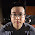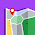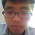## Frequent ItemSets : Apriori Algorithm and Example Part I

This is the starting for our new Tutorial Topic, "Data Mining". Apriori Algorithm is one of the classic algorithm used in Data Mining to find association rules. An initial reading to Apriori might look complex but it's not. Let me give an example and try explaining it:

Suppose we have transactions of a shopping centre as below:

Learning association rule means finding those items which were bought together most often i.e. single items, pair-wise items, triples etc.

So, as I mentioned earlier Apriori is a classic and the most basic algorithm when it comes to find association rules. A lot of resources are available over the internet which we can find, but here I will try to make it intuitive and easy.

## Algorithm:

- A two-pass algorithm which limits the need for main memory.
- One of the Key Idea behind Apriori is Monotonicity: If a set of items I appear at least s times, so does every subset J of I.

Pass 1: Read the baskets and count in main memory the occurrence/frequency of each item.

After the Pass 1, is completed, check the count for each item. And, if the count of item is more than equal to s i.e. Count(i) >= s, then the item i is frequent. Save this for next pass.

Pass 2: Read baskets again and count in main memory the occurrence/frequency of pair of items formed using the frequent items (which we got from Pass 1).

After Pass 2  end, check for the count of each pair of item and if more than equal to s, the pair if considered to be frequent, i.e. Cunt(i, j) >= s.

## Example:

We will consider few things:

- Our Support or threshold is 3.

Our Transaction Table:

Step 1: Count the occurrence of each item.

Step 2: Remember, the algorithm says, an item is considered to be frequent if it's bought more then the Support/Threshold i.e. 3. Therefore, below is the list of Frequent Singletons.

Step 3: We start making pairs out of the frequent itemsets we got in the above step.

Step 4: After getting the frequent Item Pairs, we start counting the occurrence of these pairs in the Transaction Set.

Step 5: Now again, follow the Golden Rule, and discard non-frequent paris.

Now we have a table with pair of frequent items. Suppose we want to find frequent triplets. We the above table and make all the possible combinations.

Step 6: Make combinations of triples using the frequent Item pairs.

To make triples, the rule is: IF 12 and 13 are frequent, then the triple would be 123. Similarly, if 24 and 26 then triple would be 246.

So, using the above logic and our Frequent ItemPairs table, we get the below triples:

Step 7: Get the count of the above triples (Candidates).

After, this, if we can find quartets, then we find those and count their occurrence/frequency.

If we had 123, 124, 134, 135, 234 and we wanted to generate a quartet then it would be 1234 and 1345. And after finding quartet we would have again got their count of occurrence /frequency and repeated the same also, until the Frequent ItemSet is null.

Thus, the frequent ItemSets are:

- Frequent Itemsets of Size 1: 1, 2, 4, 5, 6
- Frequent Itemsets of Size 2: 14, 24, 25, 45, 46
- Frequent Itemsets of Size 3: 245

To know more about how good the association rule formed is, i.e. calculating the confidence and  explanation of support, please click here for the Part II of this.

SHARE THIS POST:

#### 9 comments:

1.Good explanation !!

2.3.Outsource Seo
Good example to find the association rules in data mining

4.5.Thanks for this tutorial. I found here only a clear and illuminating example about the solution this algorithm would output.

I have a question about the problem I am trying to solve:
on the 'Step 7' quartets generated are 1234 and 1345, but how can I do for obtaining 1234 only (because, for my purpose, I can't build 1345 for the reason that 1345 is compose by 134,135,145,345 subsets and 145 and also 345 are not among the triples.

6.White label Seo
After reading this post, I got to know lot of new ideas.
Thanks for sharing this post.

7.Helpful ! :)

8.Really useful.. Thank you

9.very Helpful!, by the way, if using fp-growth algorithm how do you handle the repeat item like T5: 0,2,2,4,5 in this blog?